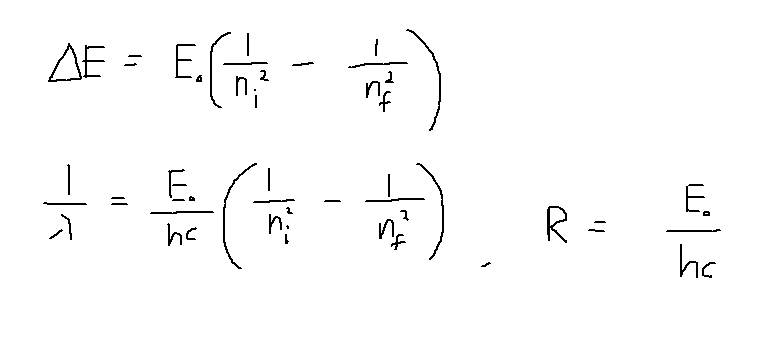# Atomic absorption spectrum

unscientific

## Homework Statement

The following photon wavelengths are observed in absorption at room temperature from an ionized atomic gas with a single electron orbiting the nucleus: λ=
13:5 nm, 11:4 nm, 10:8 nm. Use this data to determine the effective Rydberg constant
and the nuclear charge.

## The Attempt at a Solution

I know that energy is emitted/absorbed when electron transits between different energy levels.Thing is, I don't even know what the n=1 energy is, to find the rydberg constant. I can't tell what the energy levels are just from the photons absorbed, as they could be between any two levels.

## Answers and Replies

Mentor
Don't give up before you even started.
Did you convert the wavelength values to photon energies?
Which patterns do you expect in the energy spectrum?

unscientific
Don't give up before you even started.
Did you convert the wavelength values to photon energies?
Which patterns do you expect in the energy spectrum?

I expect, the gaps between energy levels to become smaller as you go higher up..

unscientific
Don't give up before you even started.
Did you convert the wavelength values to photon energies?
Which patterns do you expect in the energy spectrum?

13.5nm -> 92.1 eV
11.4nm -> 109.0 eV
10.8nm -> 115.1 eV

So

n=1 is 92.1 eV
n=2 is 109.0 eV
n=3 is 115.5 eV

Then what is the point of giving us the other 2 absorption wavelengths?

Mentor
So

n=1 is 92.1 eV
n=2 is 109.0 eV
n=3 is 115.5 eV

Then what is the point of giving us the other 2 absorption wavelengths?
Photon energies are not the energies of states!

Which photon energies (not electron energy states) do you get for a hydrogen atom? Which ratios do you have between those values? Do you see a similarity to your problem?

•1 person
unscientific
For Hydrogen:

E1 = -13.6 eV
E2 = -3.4 eV
E3 = -1.51 eV
E4 = -0.850 eV

Based on the wavelengths given, 92.1eV, 109eV, 115.5eV are differences in energy between En and E1.

The ratio of first energy level between the gas and hydrogen = Z2, where Z is the proton number of the gas.

unscientific
Photon energies are not the energies of states!

Which photon energies (not electron energy states) do you get for a hydrogen atom? Which ratios do you have between those values? Do you see a similarity to your problem?

Ok using the relation ΔE = (1- 1/n2)

First emission:
92.1 = E1(3/4)

Second emission:
109 = E1(8/9)

Third Emission:

115.5 = E1(15/16)

These ratios match, so somehow these are the emissions from the second, third and fourth energy levels.

E1 = (13.6)Z2

Solving, Z = 3 (Lithium) and E1 = 122.4eV, R = (13.6eV)/hc = 1.09*107

Last edited:
Mentor
That is correct.

R = (13.6eV)/hc = 1.09*107
I think the factor of 9 is missing here, and the last value should have units.